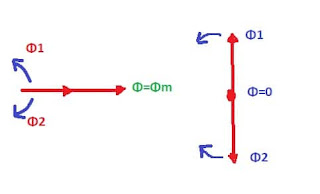# Double Revolving Field Theory

The double field revolving theory of single phase induction motor is proposed to explain this problem of no torque developed at starting and yet torque once rotated. Double revolving field theory is based on the fact that an alternating sinusoidal flux can be expressed by two revolving fluxes and each flux equal to one half of the maximum value of alternating flux Φm. i.e Φm/2 and each flux rotating at synchronous speed Ns = 120f/p, in the opposite direction of each other.

The instantaneous value of flux due to the stator current of single phase induction motor is,
Φ=Φm cosωt

Let, two rotating magnetic fluxes Φ1 and  Φ2, each flux having the magnitude of Φm/2 and rotating in the opposite direction with angular velocity ω.
Consider the two fluxes start revolving from OX axis at a time t=0, after some time t second the angle through which flux vector have rotated is at

Resolving the flux vector along x-axis and y-axis we get

X component=Φm/2* cosωt + Φm/2* cosωt =Φm cosωt

Y component=Φm/2 *sinωt - Φm/2 * sinωt =0

This resultant flux vector Φ=Φm cosωt along x-axis therefore as shown in figure alternating field can be replaced by two relating fields of half in amplitude and revolving in the opposite direction to each other at synchronous speed. here we can see that the resultant vector of two revolving flux is a stationary vector that oscillates in length with time along the x-axis.Field revolving theory
When the rotating flux vector is in phase as shown in the diagram the resultant vector is Φ=Φm, When out of phase by 180 degrees the resultant vector is Φ=0.

## Explanation of operation of single phase induction motor with revolving theory

we can explain single-phase motor operation using Double Field Revolving theory

1) Rotor at stationary

Consider case 1, that rotor is at a standstill and single-phase induction motors stator winding connected to single phase supply. Stator winding produced alternating fluxes and can be presented as a sum of two rotating fluxes Φ1 and Φ2. Each equal to Φm/2  Where Φm= maximum value of alternating flux and revolving at synchronous speed Ns in opposite direction to each other. Consider a flux Φ1 rotates in the anticlockwise direction and the other flux Φ2  rotates in the clockwise direction. flux Φ1  produced torque T1 in the anticlockwise direction and other flux Φ2  produced torque T2 in the clockwise direction. at Stationery rotor,  these two torques are equal in magnitude and opposite in direction and the net torque developed is zero. This is the reason single phase induction motor is not self starting. Read:-Why single-phase induction motor is not self-starting-reason?

2) Rotor Running

Now consider rotor is given an initial rotation by an external force. the torque due to rotating field acting in the direction of initial rotation will be more than the torque due to other rotating fields.
Hence motor will develop net positive torque in the same direction as the initial rotation and continue to rotate in the direction of initial rotation.

Rotor started, by rotating in the clockwise direction with speed N. the flux rotating in the clockwise direction called as forward rotating flux Φf and has synchronous speed Ns. and flux rotating anticlockwise direction called as a backward flux Φb having speed -Ns.

The slip in forward direction is
The rotor rotates opposite to the rotation of the backward flux. Therefore, the slip w.r.t. the backward flux will be
At standstill, speed =0  Hence Sf=Sb=1  for forward rotating flux, slip s and has a value less than 1 and for backward rotating flux, slip is 2-s and its value is greater than 1.